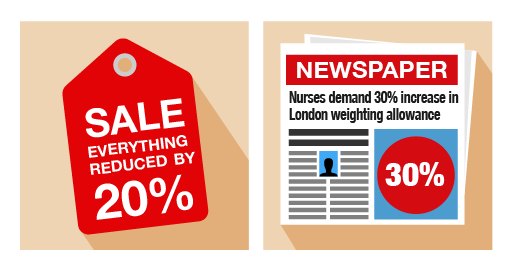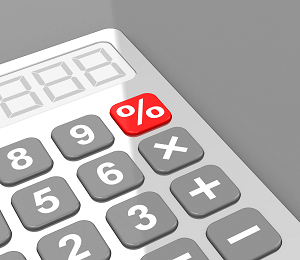Everyday maths 1

Start this free course now. Just create an account and sign in. Enrol and complete the course for a free statement of participation or digital badge if available.

Free course

# 5.1 Percentage increases and decreases

You’ll often see percentage increases and decreases in sales and pay rises.Figure 23 Increasing and decreasing percentages

## Example: Anjali’s pay rise

Anjali earns £18,000 per year. She is given a 10% pay rise. How much does she now earn?

### Method

In order to identify Anjali’s new salary, you need to find out what 10% () of £18,000 is. To do this, first you need to find out of £18,000:

18,000 ÷ 100 = 180

So of £18,000 is:

10 × 180 = 1,800

Anjali’s pay rise is £1,800, so her new salary is:

£18,000 + £1,800 = £19,800

## Example: A sale at the furniture shop

A furniture shop reduces all of its prices by 20%. How much does a £300 double bed cost in the sale?

### Method

In order to identify the new price of the double bed, you need to find out what 20% () of £300 is. To do this, first you need to find out of £300:

300 ÷ 100 = 3

So of £300 is:

20 × 3 = 60

The discount is £60, so the sale price of the double bed is:

£300 – £60 = £240

## Activity 16: Calculating percentage increases and decreases

1. You buy a car for £9,000. Its value depreciates (decreases) by 25% annually. How much will the car be worth at the end of the first year?
2. Since the start of the 21st century, the shares in the InstaBank have risen by 30%. If the price of one share was £10 in 2000, what is it worth now?

1. In order to identify how much the value of the car will decrease by, you need to find out what 25% () of £9,000 is. To do this, first you need to find out of £9,000:
• 9,000 ÷ 100 = 90
• So of £9,000 is:
• 25 × 90 = 2,250
• The car’s value depreciates by £2,250 in the first year, so the value of the car at the end of the first year will be:
• £9,000 – £2,250 = £6,750
1. It might be easier in this example to convert £10 into pence (£10 = 1,000p). In order to identify the new value of the share, you need to find out what 30% () of 1,000p is. To do this, first you need to find out of 1,000p:
• 1,000 ÷ 100 = 10
• So of 1,000p is:
• 30 × 10 = 300
• The share’s value has increased by 300p, or £3, since 2000, so the current value of the share is:
• £10 + £3 = £13

In this section you have learned how to calculate percentage increases and decreases. This will be useful when working out the value of a pay increase or how much an item will cost in a sale. You have also seen, and successfully used, two methods of calculating a percentage. There is one method that you haven’t been shown (and it’s probably the easiest!): using the percentage button on your calculator. The percentage button looks like this:Figure 24 The percentage button on a calculator

To successfully use it when calculating percentages you would enter the sum into your calculator as follows.

If you were asked to find 20% of 80, on your calculator you would input:

• 80 × 20%

This would give you the following answer:

• 80 × 20% = 16

This is by far the easiest way of calculating percentages when you have a calculator handy.

## Summary

In this section you have learned how to solve problems using percentages, and how to calculate percentage increases and decreases.

FSM_1